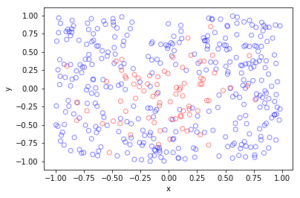## Creating a reversible Markov chain using acceptance(-rejection)

The study of Markov chains is generally the study of their long term behaviour, which, under certain conditions, is captured by them having a unique stationary distribution. Stationarity is an important property. It is, in a sense, a local property of a Markov chain.

For a Markov chain, a more global property is something called reversibility. Markov chains with this property must possess a stationary distribution, which, we see below, is an immediate consequence of reversibility. Reversible Markov chains (or processes) with discrete time1Asmussen writes in his book Applied Probability and Queues:

In this book, we use the terminology that a Markov chain has discrete time and a Markov process has continuous time (the state space may be discrete or general). However, one should note that it is equally common to let “chain” refer to a discrete state space and “process” to a general one (time may be discrete or continuous).
are the cornerstone of Markov chain Monte Carlo (MCMC) methods.

In this post we look at how a reversible Markov chain is constructed from a non-reversible (but irreducible) Markov chain by introducing an acceptance-rejection step. This post complements another post I wrote on the Metropolis-Hastings algorithm.

## Reversibility

A Markov process on state space $$\mathbb{X}$$ with kernel (matrix) $$K$$ is (time) reversible with respect to the distribution $$\mu$$ if the following holds

$$\mu(x)K(x,y) = \mu (y) K(y,x)\quad x,y\in\mathbb{X}\,.$$

This reversibility condition is also called the detailed balance equation. If this condition is met, then the Markov process will have a stationary distribution $$\mu$$. By summing over $$x$$, we can verify this because we obtain

$$\sum_{x\in\mathbb{X}}\mu(x)K(x,y) =\mu(y)\sum_{x\in\mathbb{X}} K(y,x)=\mu(y)\,.$$

This is just the balance equation, often written as $$\mu=K\mu$$, which says that the transition kernel $$K$$ has a stationary distribution $$\mu$$.

## First Markov chain

We consider a Markov chain defined on a finite state space $$\mathbb{X}$$. If the Markov chain is at state $$x\in \mathbb{X}$$, it visits another state $$y\in \mathbb{X}$$ with the probability $$J(x,y)$$. This is a simple time-homogeneous finite Markov chain.

### Irreducibility

For our Markov chain, we assume that every state $$x$$ in $$\mathbb{X}$$ where $$\pi(x)>0$$ is reachable with positive probability in a single step. This implies the easy-to-achieve condition $$J(x,y)>0$$ where $$\pi(x)>0$$ for all points $$x,y \in \mathbb{X}$$. This requirement is a stronger form of irreducibility.

## Creating a new Markov chain with acceptance

We create a new Markov chain by introducing an acceptance step. For the Markov chain with kernel $$J$$, we assume that each time step, after choosing the jump direction but before jumping, a biased coin is flipped . The success probability $$\alpha(x,y)$$ depends on the current position $$x\in \mathbb{X}$$ and the (potential) next position $$y\in \mathbb{X}$$.

### Transition kernel

For our new Markov chain, we can quickly reason the transition kernel $$M$$. We first look at the off-diagonal elements of the kernel (matrix) $$M$$. To go from state $$x$$ and to another state $$y\neq x$$, the probability is simply

$$M(x,y) = \alpha(x,y) J(x,y), \quad x\neq y\,.$$

The transition matrix $$M$$ needs to be stochastic, so the rows sum to one, so $$\sum_{y\in\mathbb{X}}M(x,y)=1$$. That gives us the diagonal elements of $$M$$, although their exact form is not needed to show reversibility.

### $$\alpha(x,y)$$ needs a symmetric function $$s(x,y)$$

For reversibility, we essentially just need to swap rows and columns. In that case, clearly we only need to look at the off-diagonal entries, which implies the requirement

$$\pi(x)M(x,y) = \pi (y) M(y,x)\quad x\neq\,.$$

Both both sides are symmetric in $$x$$ and $$y$$, meaning they are equal to some non-negative symmetric $$s(x,y)=s(y,x)$$. Looking at the right-hand side, we get

\begin{aligned}\pi(y) M(y,x)&=\pi(y)J(y,x) \alpha(y,x)\\ &= s(y,x)\,.\end{aligned}

This implies that the function $$\alpha$$ is a non-negative function such that $$\alpha\leq 1$$, to ensure it’s a probability, with the form

$$\alpha(x,y)=\frac{s(x,y)}{\pi(x)J(x,y)}\,.$$

The only task remaining now is to choose a reasonable symmetric function $$s$$ such that $$\alpha\leq 1$$, ensuring $$\alpha$$ is a probability. Of course, our choice for the symmetric $$s$$ should also be a function of the stationary distribution and the underlying kernel $$J$$.

## Examples

I’ll give two principal examples of the symmetric function $\latex s(x,y)$. Working in reverse chronological order, I’ll give the simpler of the two examples first.

### $$s(x,y)$$ of Barker

A somewhat natural example is

$$s(x,y) = \frac{\pi(x)J(x,y)\pi(y)J(y,x)}{\pi(x)J(x,y)+\pi(y)J(y,x)}\,.$$

This is clearly a symmetric function, which only has terms $$pi$$ and $$M$$. The acceptance probability becomes

$$\alpha(x,y) = \frac{\pi(y)J(y,x)}{\pi(x)J(x,y)+\pi(y)J(y,x)}\,.$$

A.A. Barker proposed this function in a 1965 paper as part of his PhD work in mathematical physics at the University of Adelaide. Barker had been inspired by a previous 1953 paper, which brings us to the next example.

### $$s(x,y)$$ of Metropolis(-Rosenbluth-Rosenbluth-Teller-Teller)-Hastings

The now most important example is

$$s(x,y) = \min[\pi(x)J(x,y),\pi(y)J(y,x)]\,.$$

We can see that this is symmetric function.

The acceptance probability becomes

$$\alpha(x,y) = \min[1,\frac{\pi(y)J(y,x)}{\pi(x)J(x,y)}]\,.$$

This example is very famous in the world of Markov chain Monte Carlo methods. It is the main part of the so-called the Metropolis-Hastings algorithm, which comes from a 1953 paper by Nicholas Metropolis, Arianna W. Rosenbluth, Marshall Rosenbluth, Augusta H. Teller, and Edward Teller (two husband-wife pairs), who looked at a special case, and a 1970 paper by W.K. Hastings, who generalized the method.

### Articles

##### Historical

The two important historical papers that gave the two examples for $$s(x,y)$$ are:

• 1965 – Barker – Monte Carlo calculations of the radial distribution functions for a proton-electron plasma;
• 1953 – Metropolis, Rosenbluth, Rosenbluth, Teller, Teller – Equation of state calculations by fast computing machines.

This paper is also important:

• 1970 – Hastings – Monte Carlo sampling methods using Markov chains and their applications.
##### Introductory

Hastings algorithm. An good explanatory article about the Metropolis-Hastings algorithm is

• 1995 – Chib and Greenberg – Understanding the Metropolis-Hastings Algorithm.

I also recommend:

• 2015 – Minh and Minh – Understanding the Hastings Algorithm;
• 2016 – Robert – The Metropolis-Hastings algorithm.
• 2017 – Gonçalves, Łatuszyński, Roberts – Barker’s algorithm for Bayesian inference with intractable likelihoods.
##### History

The history of this and related methods are described in the following article:

• 2011 – Robert and Casella – A Short History of Markov Chain Monte Carlo: Subjective Recollections from Incomplete Data.

Incidentally, the first author, Christian P. Robert, writes on his blog about Markov chain Monte Carlo methods, such as the Metropolis-Hastings algorithm, as well as many other topics.

### Books

Modern books on stochastic simulations and Monte Carlo methods will often detail this method. For example, see the Handbook of Monte Carlo Methods (Section 6.1) by Kroese, Taimre and Botev. The book Stochastic Simulation: Algorithms and Analysis by Asmussen and Glynn also covers the method in Chapter XIII, Section 3. (For my remark on reversibility, see Remark 3.2 in Asmussen and Glynn.) There is also the book Monte Carlo Strategies in Scientific Computing by Liu; see Chapter 5.

### Websites

The web abounds with pages dedicated to explaining Markov chain Monte Carlo methods with the focus typically on the Metropolis-Hastings algorithm. I would try this following one because it gives a detailed explanation on how the Metropolis-Hastings algorithm works:

• https://gregorygundersen.com/blog/2019/11/02/metropolis-hastings/

## The acceptance(-rejection) method for simulating random variables

In a previous post, I covered a simple but much used method for simulating random variables or, rather, generating random variates. To simulate a random variable, the method requires, in an easy fashion, calculating the inverse of its cumulative distribution function. But you cannot always do that.

In lieu of this, the great John von Neumann wrote in a 1951 paper that you can sample a sequence of values from another probability distribution, accepting only the values that meet a certain condition based on this other distribution and the desired distribution, while rejecting all the others. The accepted values will follow the desired probability distribution. This method of simulation or sampling is called the rejection method, the acceptance method, and it has even the double-barrelled name the acceptance-rejection (AR) method.

## Details

Let $$X$$ be a continuous random variable with a (probability) density $$p(x)$$, which is the derivative of its cumulative probability distribution $$P(X\leq x)$$. The density $$p(x)$$ corresponds to the desired or target distribution from which we want to sample. For whatever reason, we cannot directly simulate the random variable $$X$$. (Maybe we cannot use the inverse method because $$P(X\leq x)$$ is too complicated.)

The idea that von Newman had was to assume that we can easily simulate another random variable, say, $$Y$$ with the (probability) density $$q(x)$$. The density $$q(x)$$ corresponds to a proposal distribution that we can sample (by using, for example, the inverse method).

Now we further assume that there exists some finite constant $$M>0$$ such that we can bound $$p(x)$$ by $$Mq(x)$$, meaning

$$p(x) \leq M q(x), \text{ for all } x .$$

Provided this, we can then sample the random variable $$Y$$ and accept a value of it (for a value of $$X$$) with probability

$$\alpha = \frac{p(Y)}{Mq(Y)}.$$

If the sampled value of $$Y$$ is not accepted (which happens with probability $$1-\alpha$$), then we must repeat this random experiment until a sampled value of $$Y$$ is accepted.

## Algorithm

We give the pseudo-code for the acceptance-rejection method suggested by von Neumann.

Random variable $$X$$ with density $$p(x)$$

1. Sample a random variable $$Y$$ with density $$q(x)$$, giving a sample value $$y$$.
2. Calculate the acceptance probability $$\alpha = \frac{p(y)}{Mq(y)}$$.
3. Sample a uniform random variable $$U\sim U(0,1)$$, giving a sample value $$u$$.
4. Return the value $$y$$ (for the value of $$X$$) if $$u\leq \alpha$$, otherwise go to Step 1 and repeat.

As covered in a previous post, Steps 3 and 4 are equivalent to accepting the value $$y$$ with probability $$\alpha$$.

## Point process application

In the context of point processes, this method is akin to thinning point processes independently. This gives a gives a method for positioning points non-uniformly by first positioning the points uniformly. The points are then thinned based on the desired intensity function. As I covered in a previous post, this is one way to simulate an inhomogeneous (or nonhomogeneous) Poisson point process.

## Efficiency

Of course the number of experiment runs (Steps 1 to 3) until acceptance is a geometric variable with parameter $$\alpha$$. On average the acceptance(-rejection) method will take $$1/\alpha$$ number of simulations to sample one value of the random $$X$$ of the target distribution. The key then is to make the proposal density $$q(x)$$ as small as possible (and adjust $$M$$ accordingly), while still keeping the inequality $$p(x) \leq M q(x)$$.

## Higher dimensions

The difficulty of the acceptance(-rejection) method is finding a good proposal distribution such that the product $$Mq(x)$$ is not much larger than the target density $$p(x)$$. In one-dimension, this can be often done, but in higher dimensions this becomes increasingly difficult. Consequently, this method is typically not used in higher dimensions.

Another approach with an acceptance step is the Metropolis-Hastings method, which is the quintessential Markov chain Monte Carlo (MCMC) method. This method and its cousins have become exceedingly popular, as they give ways to simulate collections of dependent random variables that have complicated (joint) distributions.

The original paper where the acceptance(-rejection) method appears (on page 769 in the right-hand column) is:

• von Neumann, Various techniques used in connection with random digits, 1951.

The usual books on stochastic simulations and Monte Carlo methods will detail this method. For example, see the book by Devroye (Section II.3) or the more recent Handbook of Monte Carlo Methods (Section 3.1.5) by Kroese, Taimre and Botev. The book Stochastic Simulation: Algorithms and Analysis by Asmussen and Glynn also covers the method in Section 2b.

Other books include those by Fishman (Section 8.5) and Gentle (Section 4.5) respectively.

## Thinning point processes

One way to create new point processes is to apply thinning to a point process. As I mentioned in a previous post on point process operations, thinning is a random operation applied to the points of an underlying point process, where the points are thinned (or removed) or retained (or kept) according to some probabilistic rule. Both the thinned and retained points form two separate point processes, but one usually focuses on the retained points. Given an underlying point process, the nature of the thinning rule will result in different types of point processes.

As I detailed in the Applications section below, thinning can be used to simulate an inhomogeneous Poisson point process, as I covered in another post.

## Thinning types

Thinning can be statistically independent or dependent, meaning that the probability of thinning any point is either independent or dependent of thinning other points. The more tractable case is statistically independent thinning, which is the thinning type covered here. We can further group this thinning into two types based on whether the thinning rule depends on the locations of the point. (I use the word location, instead of point, to refer to where a point of a point process is located on the underlying mathematical space on which the point process is defined.)

##### Spatially independent thinning

The simplest thinning operation is one that does not depend on point locations. This thinning is sometimes referred to as $$p$$-thinning, where the constant $$p$$ has the condition $$0\leq p \leq 1$$ because it is the probability of thinning a single point. Simply put, the probability of a point being thinned does not depend on the point locations.

Example

We can liken the thinning action to flipping a biased coin with probability of $$p$$ for heads (or tails) for each point of the underlying point process, and then removing the point if a head (or tails) occurs. If there were a constant number $$n$$ of points of the underlying point process, then the number of thinned (or retained) points will form a binomial random variable with parameters $$n$$ and $$p$$ (or $$1-p$$).

Simulation

Simulating this thinning operation is rather straightforward. Given a realization of a point process, for each point, simply generate or simulate a uniform random variable on the interval $$(0,1)$$, and if this random variable is less than $$p$$, remove the point. (This is simply sampling a Bernoulli distribution, which is covered in this post.)

In the code section below, I have shown how this thinning operation is implemented.

##### Spatially dependent thinning

To generalize the idea of $$p$$-thinning, we can simply require that the thinning probability of any point depends on its location $$x$$, which gives us the concept of $$p(x)$$-thinning. (I write a single $$x$$ to denote a point on the plane, that is $$x\in \mathbb{R}^2$$, instead of writing, for example, the $$x$$ and $$y$$ and coordinates separately.) More precisely, the probability of thinning a point is given by a function $$p(x)$$ such that $$0 \leq p(x)\leq 1$$, but all point thinnings occur independently of each other. In other words, this is a spatially dependent thinning that is statistically independent.

Example

I’ll illustrate the concept of (statistically independent) spatially dependent thinning with a somewhat contrived example. We assume that the living locations of all the people in the world form a point process on a (slightly squashed) sphere. Let’s say that Earth has become overpopulated, particularly in the Northern Hemisphere, so we decide to randomly choose people and send them off to another galaxy, but we do it based on how far they live from the North Pole. The thinning rule could be, for example, $$p(x)= \exp(- |x|^2/s^2)$$, where $$|x|$$ is the distance to the North Pole and $$s>0$$ is some constant for distance scaling.

Put another way, a person at location $$x$$ flips a biased coin with the probability of heads being equal to $$p(x)=\exp(- |x|^2/s^2)$$. If a head comes up, then that person is removed from the planet. With the maximum of $$p(x)$$ is at the North Pole, we can see that the lucky (or unlucky?) people in countries like Australia, New Zealand (or Aotearoa), South Africa, Argentina and Chile, are more likely not to be sent off (that is, thinned) into the great unknown.

For people who live comparable distances from the North Pole, the removal probabilities are similar in value, yet the events of being remove remain independent. For example, the probabilities of removing any two people from the small nation Lesotho are similar in value, but these two random events are still completely independent of each other.

Simulation

Simulating a spatially dependent thinning is just slightly more involved than the spatially independent case. Given a realization of a point process, for each point at, say, $$x$$, simply generate or simulate a uniform random variable on the interval $$(0,1)$$, and if this random variable is less than $$p(x)$$, remove the point.

In the code section, I have shown how this thinning operation is implemented with an example like the above one, but on a rectangular region of Cartesian space. In this setting, the maximum of $$p(x)$$ is at the origin, resulting in more points being thinned in this region.

## Thinning a Poisson point process

Perhaps not surprisingly, under the thinning operation the Poisson point process exhibits a closure property, meaning that a Poisson point process thinned in a certain way gives another Poisson point process.  More precisely, if the thinning operation is statistically independent, then the resulting point process formed from the retained points is also a Poisson point process, regardless if it is spatially independent or dependent thinning. The resulting intensity (interpreted as the average density of points) of this new Poisson point process has a simple expression.

##### Homogeneous case

For a spatially independent $$p$$-thinning, if the original (or underlying) Poisson point process is homogeneous with intensity $$\lambda$$, then the point process formed from the retained points is a homogeneous Poisson point process with intensity $$\lambda$$.  (There are different ways to prove this, but you can gain some intuition behind the proof by conditioning on the Poisson number of points and then applying the total law of probability. Using generating functions helps.)

##### Inhomogeneous case

More generally, if we apply a spatially dependent $$p(x)$$-thinning to a  Poisson point process has a intensity $$\lambda$$, then the retained points form a  an inhomogeneous or nonhomogeneous Poisson point process with $$\lambda p(x)$$,  due to the spatial dependence in the thinning function $$p(x)$$. This gives a way to simulate such Poisson point processes, which I’ll cover in another post.

##### Splitting

We can see by symmetry that if we look at the thinned points, then the resulting point process is also a Poisson point process, but with intensity $$(1-p(x))\lambda$$. The retained and thinned points both form Poisson point processes, but what is really interesting is these two point processes are independent of each other.  This means that any random configuration that occurs among the retained points is independent of any configurations among the thinned points.

This ability to split a Poisson point processes into independent ones is sometimes called the splitting property.

## Applications

Thinning point processes has the immediate application of creating new point processes. It can also be used to randomly generate two point processes from one. In network applications, a simple example is using the thinning procedure to model random sleep schemes in wireless networks, where random subsets of the network have been powered down.

Perhaps the most useful application of thinning is creating point processes with spatially-dependent intensities such that of an inhomogeneous Poisson point process. In another post I give details on how to simulate this point process.  In this setting, the thinning operation essentially is acceptance(-rejection) sampling, which I will cover in a future post.

## Code

All code from my posts, as always, can be found on the my GitHub repository. The code for this post is located here.

##### Spatially independent thinning

I have implemented in code the simple $$p$$-thinning operation applied to a Poisson point process on a rectangle, but in theory any point process can be used for the underlying point process that is thinned.

MATLAB

%Simulation window parameters
xMin=-1;xMax=1;
yMin=-1;yMax=1;
xDelta=xMax-xMin;yDelta=yMax-yMin; %rectangle dimensions
areaTotal=xDelta*yDelta; %area of rectangle

%Point process parameters
lambda=100; %intensity (ie mean density) of the Poisson process

%Thinning probability parameters
sigma=1;
p=0.25; %thinning probability

%Simulate Poisson point process
numbPoints=poissrnd(areaTotal*lambda);%Poisson number of points
xx=xDelta*(rand(numbPoints,1))+xMin;%x coordinates of Poisson points
yy=xDelta*(rand(numbPoints,1))+yMin;%y coordinates of Poisson points

%Generate Bernoulli variables (ie coin flips) for thinning
booleThinned=rand(numbPoints,1)&amp;amp;gt;p; %points to be thinned
booleRetained=~booleThinned; %points to be retained

%x/y locations of thinned points
xxThinned=xx(booleThinned); yyThinned=yy(booleThinned);
%x/y locations of retained points
xxRetained=xx(booleRetained); yyRetained=yy(booleRetained);

%Plotting
plot(xxRetained,yyRetained,'bo'); %plot retained points
hold on; plot(xxThinned,yyThinned,'ro'); %plot thinned points
xlabel('x');ylabel('y');


R

#Simulation window parameters
xMin=-1;xMax=1;
yMin=-1;yMax=1;
xDelta=xMax-xMin;yDelta=yMax-yMin; #rectangle dimensions
areaTotal=xDelta*yDelta;

#Point process parameters
lambda=100; #intensity (ie mean density) of the Poisson process

#Thinning probability
p=0.25;

#Simulate a Poisson point process
numbPoints=rpois(1,areaTotal*lambda);#Poisson number of points
xx=xDelta*runif(numbPoints)+xMin;#x coordinates of Poisson points
yy=xDelta*runif(numbPoints)+yMin;#y coordinates of Poisson points

#Generate Bernoulli variables (ie coin flips) for thinning
booleThinned=runif(numbPoints)&amp;amp;gt;p; #points to be thinned
booleRetained=!booleThinned; #points to be retained

#x/y locations of thinned points
xxThinned=xx[booleThinned]; yyThinned=yy[booleThinned];
#x/y locations of retained points
xxRetained=xx[booleRetained]; yyRetained=yy[booleRetained];

#Plotting
par(pty="s")
plot(xxRetained,yyRetained,'p',xlab='x',ylab='y',col='blue'); #plot retained points
points(xxThinned,yyThinned,col='red'); #plot thinned points



Of course, as I have mentioned before, simulating a spatial point processes in R is even easier with the powerful spatial statistics library spatstat.  With this library, thinning can be done in quite a general way by using the function rthin.

Python

import numpy as np; #NumPy package for arrays, random number generation, etc
import matplotlib.pyplot as plt

#Simulation window parameters
xMin=-1;xMax=1;
yMin=-1;yMax=1;
xDelta=xMax-xMin;yDelta=yMax-yMin; #rectangle dimensions
areaTotal=xDelta*yDelta;

#Point process parameters
lambda0=100; #intensity (ie mean density) of the Poisson process

#Thinning probability
p=0.25;

#Simulate a Poisson point process
numbPoints = np.random.poisson(lambda0*areaTotal);#Poisson number of points
xx = np.random.uniform(0,xDelta,((numbPoints,1)))+xMin;#x coordinates of Poisson points
yy = np.random.uniform(0,yDelta,((numbPoints,1)))+yMin;#y coordinates of Poisson points

#Generate Bernoulli variables (ie coin flips) for thinning
booleThinned=np.random.uniform(0,1,((numbPoints,1)))&amp;amp;gt;p; #points to be thinned
booleRetained=~booleThinned; #points to be retained

#x/y locations of thinned points
xxThinned=xx[booleThinned]; yyThinned=yy[booleThinned];
#x/y locations of retained points
xxRetained=xx[booleRetained]; yyRetained=yy[booleRetained];

#Plotting
plt.scatter(xxRetained,yyRetained, edgecolor='b', facecolor='none', alpha=0.5 );
plt.scatter(xxThinned,yyThinned, edgecolor='r', facecolor='none', alpha=0.5 );
plt.xlabel("x"); plt.ylabel("y");
plt.show();

##### Spatially dependent thinning

I have implemented in code a $$p(x)$$-thinning operation with the function $$p(x)=\exp(-|x|^2/s^2)$$, where $$|x|$$ is the Euclidean distance from $$x$$ to the origin. This small changes means that in the code there will be a vector or array of $$p$$ values instead of a single $$p$$ value in the section where the uniform random variables are generated and compared said $$p$$ values.  (Lines 24, 26 and 28 respectively in the MATLAB, R and Python code presented below.)

Again, I have applied thinning to a Poisson point process on a rectangle, but in theory any point process can be used for the underlying point process.

MATLAB

%Simulation window parameters
xMin=-1;xMax=1;
yMin=-1;yMax=1;
xDelta=xMax-xMin;yDelta=yMax-yMin; %rectangle dimensions
areaTotal=xDelta*yDelta; %area of rectangle

%Point process parameters
lambda=100; %intensity (ie mean density) of the Poisson process

%Thinning probability parameters
sigma=0.5; %scale parameter for thinning probability function
%define thinning probability function
fun_p=@(s,x,y)(exp(-(x.^2+y.^2)/s^2));

%Simulate Poisson point process
numbPoints=poissrnd(areaTotal*lambda);%Poisson number of points
xx=xDelta*(rand(numbPoints,1))+xMin;%x coordinates of Poisson points
yy=xDelta*(rand(numbPoints,1))+yMin;%y coordinates of Poisson points

%calculate spatially-dependent thinning probabilities
p=fun_p(sigma,xx,yy);

%Generate Bernoulli variables (ie coin flips) for thinning
booleThinned=rand(numbPoints,1)&amp;amp;gt;p; %points to be thinned
booleRetained=~booleThinned; %points to be retained

%x/y locations of thinned points
xxThinned=xx(booleThinned); yyThinned=yy(booleThinned);
%x/y locations of retained points
xxRetained=xx(booleRetained); yyRetained=yy(booleRetained);

%Plotting
plot(xxRetained,yyRetained,'bo'); %plot retained points
hold on; plot(xxThinned,yyThinned,'ro'); %plot thinned points
xlabel('x');ylabel('y');


R

#Simulation window parameters
xMin=-1;xMax=1;
yMin=-1;yMax=1;
xDelta=xMax-xMin;yDelta=yMax-yMin; #rectangle dimensions
areaTotal=xDelta*yDelta;

#Point process parameters
lambda=100; #intensity (ie mean density) of the Poisson process

#Thinning probability parameters
sigma=0.5; #scale parameter for thinning probability function
#define thinning probability function
fun_p &amp;amp;lt;- function(s,x,y) {
exp(-(x^2 + y^2)/s^2);
}

#Simulate a Poisson point process
numbPoints=rpois(1,areaTotal*lambda);#Poisson number of points
xx=xDelta*runif(numbPoints)+xMin;#x coordinates of Poisson points
yy=xDelta*runif(numbPoints)+yMin;#y coordinates of Poisson points

#calculate spatially-dependent thinning probabilities
p=fun_p(sigma,xx,yy);

#Generate Bernoulli variables (ie coin flips) for thinning
booleThinned=runif(numbPoints)&amp;amp;lt;p; #points to be thinned
booleRetained=!booleThinned; #points to be retained

#x/y locations of thinned points
xxThinned=xx[booleThinned]; yyThinned=yy[booleThinned];
#x/y locations of retained points
xxRetained=xx[booleRetained]; yyRetained=yy[booleRetained];

#Plotting
par(pty="s")
plot(xxRetained,yyRetained,'p',xlab='x',ylab='y',col='blue'); #plot retained points
points(xxThinned,yyThinned,col='red'); #plot thinned points


Again, use the spatial statistics library spatstat, which has the function rthin.

Python

import numpy as np; #NumPy package for arrays, random number generation, etc
import matplotlib.pyplot as plt

#Simulation window parameters
xMin=-1;xMax=1;
yMin=-1;yMax=1;
xDelta=xMax-xMin;yDelta=yMax-yMin; #rectangle dimensions
areaTotal=xDelta*yDelta;

#Point process parameters
lambda0=100; #intensity (ie mean density) of the Poisson process

#Thinning probability parameters
sigma=0.5; #scale parameter for thinning probability function
#define thinning probability function
def fun_p(s, x, y):
return np.exp(-(x**2+y**2)/s**2);

#Simulate a Poisson point process
numbPoints = np.random.poisson(lambda0*areaTotal);#Poisson number of points
xx = np.random.uniform(0,xDelta,((numbPoints,1)))+xMin;#x coordinates of Poisson points
yy = np.random.uniform(0,yDelta,((numbPoints,1)))+yMin;#y coordinates of Poisson points

#calculate spatially-dependent thinning probabilities
p=fun_p(sigma,xx,yy);

#Generate Bernoulli variables (ie coin flips) for thinning
booleThinned=np.random.uniform(0,1,((numbPoints,1)))&amp;amp;gt;p; #points to be thinned
booleRetained=~booleThinned; #points to be retained

#x/y locations of thinned points
xxThinned=xx[booleThinned]; yyThinned=yy[booleThinned];
#x/y locations of retained points
xxRetained=xx[booleRetained]; yyRetained=yy[booleRetained];

#Plotting
plt.scatter(xxRetained,yyRetained, edgecolor='b', facecolor='none', alpha=0.5 );
plt.scatter(xxThinned,yyThinned, edgecolor='r', facecolor='none', alpha=0.5 );
plt.xlabel("x"); plt.ylabel("y");
plt.show();


### Results

In the plotted results, the blue and red circles represent respectively the retained and thinned points.

##### Spatially independent thinning

For these results, I used a thinning probability $$p=0.25$$, which means that roughly a quarter of the points will be thinned, so on average the ratio of blue to red circles is three to one.

MATLAB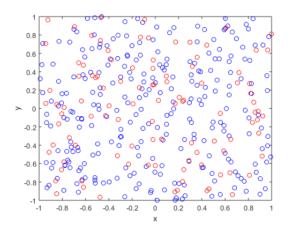R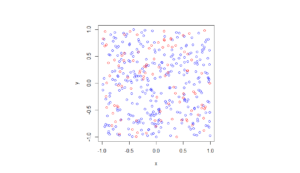Python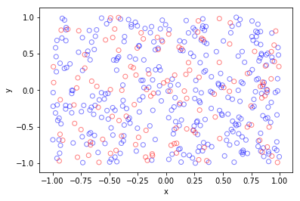##### Spatially dependent thinning

Observe how there are more thinned points (that is, red circles) near the origin, which is of course where the thinning function $$p(x)=\exp(-|x|^2/s^2)$$ attains its maximum.

MATLAB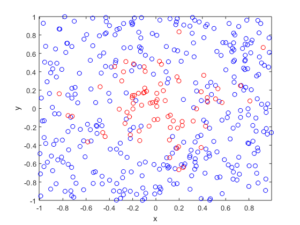R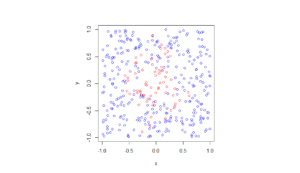Python